# Variations without repetition

Find out how many different ways you can choose k items from n items set. With/without repetition, with/without order.

(n)
(k)

# A bit of theory - foundation of combinatorics

## Variations

A variation of the k-th class of n elements is an ordered k-element group formed from a set of n elements. The elements are not repeated and depend on the order of the group's elements (therefore arranged).

The number of variations can be easily calculated using the combinatorial rule of product. For example, if we have the set n = 5 numbers 1,2,3,4,5 and we have to make third-class variations, their V3 (5) = 5 * 4 * 3 = 60.
${V}_{k}\left(n\right)=n\left(n-1\right)\left(n-2\right)\mathrm{.}\mathrm{.}\mathrm{.}\left(n-k+1\right)=\frac{n!}{\left(n-k\right)!}$
n! we call the factorial of the number n, which is the product of the first n natural numbers. The notation with the factorial is only clearer, equivalent. For calculations, it is fully sufficient to use the procedure resulting from the combinatorial rule of product.

## Permutations

The permutation is a synonymous name for a variation of the nth class of n-elements. It is thus any n-element ordered group formed of n-elements. The elements are not repeated and depend on the order of the elements in the group.
$P\left(n\right)=n\left(n-1\right)\left(n-2\right)\mathrm{.}\mathrm{.}\mathrm{.}1=n!$
A typical example is: We have 4 books, and in how many ways can we arrange them side by side on a shelf?

## Variations with repetition

A variation of the k-th class of n elements is an ordered k-element group formed of a set of n elements, wherein the elements can be repeated and depends on their order. A typical example is the formation of numbers from the numbers 2,3,4,5, and finding their number. We calculate their number according to the combinatorial rule of the product:
${V}_{k}^{\mathrm{\prime }}\left(n\right)=n\cdot n\cdot n\cdot n\mathrm{.}\mathrm{.}\mathrm{.}n={n}^{k}$

## Permutations with repeat

A repeating permutation is an arranged k-element group of n-elements, with some elements repeating in a group. Repeating some (or all in a group) reduces the number of such repeating permutations.
${P}_{{k}_{1}{k}_{2}{k}_{3}\mathrm{.}\mathrm{.}\mathrm{.}{k}_{m}}^{\mathrm{\prime }}\left(n\right)=\frac{n!}{{k}_{1}!{k}_{2}!{k}_{3}!\mathrm{.}\mathrm{.}\mathrm{.}{k}_{m}!}$
A typical example is to find out how many seven-digit numbers formed from the numbers 2,2,2, 6,6,6,6.

## Combinations

A combination of a k-th class of n elements is an unordered k-element group formed from a set of n elements. The elements are not repeated, and it does not matter the order of the group's elements. In mathematics, disordered groups are called sets and subsets. Their number is a combination number and is calculated as follows:
${C}_{k}\left(n\right)=\left(\genfrac{}{}{0px}{}{n}{k}\right)=\frac{n!}{k!\left(n-k\right)!}$
A typical example of combinations is that we have 15 students and we have to choose three. How many will there be?

## Combinations with repeat

Here we select k element groups from n elements, regardless of the order, and the elements can be repeated. k is logically greater than n (otherwise, we would get ordinary combinations). Their count is:
${C}_{k}^{\mathrm{\prime }}\left(n\right)=\left(\genfrac{}{}{0px}{}{n+k-1}{k}\right)=\frac{\left(n+k-1\right)!}{k!\left(n-1\right)!}$
Explanation of the formula - the number of combinations with repetition is equal to the number of locations of n − 1 separators on n-1 + k places. A typical example is: we go to the store to buy 6 chocolates. They offer only 3 species. How many options do we have? k = 6, n = 3.

## Foundation of combinatorics in word problems

• Hockey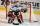The hockey match ended 8:2. How many different matches could be?
• Combinatorics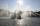The city has 7 fountains. Works only 6. How many options are there that can squirt ?
• Hockey game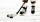In the hockey game was made six goals. Czech played against Finland. Czechs won 4:2. In what order to fall goals? How many game sequences were possible during the game?
• You have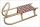You have 4 reindeer and you want to have 3 fly your sleigh. You always have your reindeer fly in a single-file line. How many different ways can you arrange your reindeer?
• CombinationsFrom how many elements we can create 990 combinations 2nd class without repeating?
• Five letters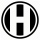How many ways can five letters be arranged?
• Two groupsThe group of 10 girls should be divided into two groups with at least 4 girls in each group. How many ways can this be done?
• Three students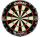Three students independently try to solve the problem. The first student will solve a similar problem with a probability of 0.6, the second student will solve at a probability of 0.55, and the third will solve at a probability of 0.04. The problem is reso
• DigitsWrite the smallest and largest 2-digit natural number.
• Subsets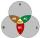How many are all subsets of set C = (97, 67, 66, 18, 59, 64)?
• Triangles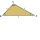Five sticks with a length of 2,3,4,5,6 cm. How many ways can you choose three sticks to form three sides of a triangle?
• Divisible by fiveHow many different three-digit numbers divisible by five can we create from the digits 2, 4, 5? The digits can be repeated in the created number.
• Probability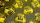What are the chances that in the lottery, in which the numbers are drawn 5 of 50, you win the first prize?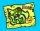There are four roads from city A to city B. There are five roads from city B to city C. How many different routes can we take from city A to city C via city B?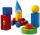3 children pulled 12 different toys from a box. Many ways can be divided toys so that each child had at least one toy?How many different combinations can we choose if there are 3 soups, 5 kinds of main dish and 2 desserts in the dining room?